Download Presentation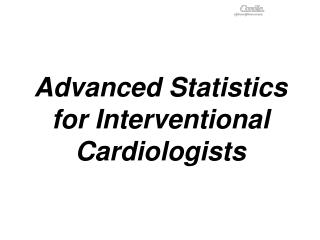Advanced Statistics for Interventional Cardiologists

# Advanced Statistics for Interventional Cardiologists - PowerPoint PPT Presentation

Advanced Statistics for Interventional Cardiologists. What you will learn. 1 st day. 2 nd day. Introduction Basics of multivariable statistical modeling Advanced linear regression methods Hands-on session: linear regression Bayesian methodsI am the owner, or an agent authorized to act on behalf of the owner, of the copyrighted work described.
Download Presentation## Advanced Statistics for Interventional Cardiologists

An Image/Link below is provided (as is) to download presentation

Download Policy: Content on the Website is provided to you AS IS for your information and personal use and may not be sold / licensed / shared on other websites without getting consent from its author.While downloading, if for some reason you are not able to download a presentation, the publisher may have deleted the file from their server.

- - - - - - - - - - - - - - - - - - - - - - - - - - E N D - - - - - - - - - - - - - - - - - - - - - - - - - -
Presentation Transcript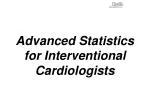### Advanced Statistics for Interventional Cardiologists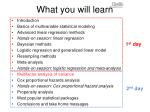What you will learn

1st day

2ndday

Introduction

Basics of multivariable statistical modeling

Advanced linear regression methods

Hands-on session: linear regression

Bayesian methods

Logistic regression and generalized linear model

Resampling methods

Meta-analysis

Hands-on session: logistic regression and meta-analysis

Multifactor analysis of variance

Cox proportional hazards analysis

Hands-on session: Cox proportional hazard analysis

Propensity analysis

Most popular statistical packages

Conclusions and take home messages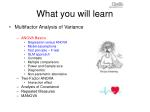What you will learn
• Multifactor Analysis of Variance
• ANOVA Basics
• Regression versus ANOVA
• Model assumptions
• Test principle – F-test
• GLM approach
• Contrasts
• Multiple comparisons
• Power and Sample size
• Diagnostics
• Non-parametric alternative
• Two-Factor ANOVA
• Interaction effect
• Analysis of Covariance
• Repeated Measures
• MANOVA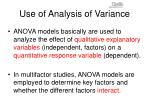Use of Analysis of Variance
• ANOVA models basically are used to analyze the effect of qualitative explanatory variables (independent, factors) on a quantitative response variable (dependent).
• In multifactor studies, ANOVA models are employed to determine key factors and whether the different factors interact.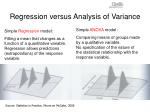Regression versus Analysis of Variance

Simple ANOVA model :

Comparing means of groups made by a qualitative variable. No specification of the nature of the statistical relationship with the response variable.

Simple Regression model:

Fitting a mean that changes as a function of a quantitative variable. Regression allows predictions (extrapolations) of the response variable.

Source: Statistics in Practice, Moore en McCabe, 2006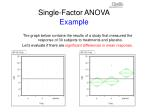Single-Factor ANOVAExample

The graph below contains the results of a study that measured the response of 30 subjects to treatments and placebo.

Let’s evaluate if there are significant differences in mean response.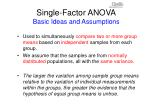Single-Factor ANOVABasic Ideas and Assumptions
• Used to simultaneously compare two or more group means based on independent samples from each group.
• We assume that the samples are from normally distributed populations, all with the same variance.
• The larger the variation among sample group means relative to the variation of individual measurements within the groups, the greater the evidence that the hypothesis of equal group means is untrue.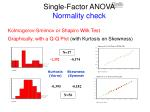N=17

-1,392

-0,174

Kurtosis

(Vorm)

Skewness

(Symmet

-0,395

-0,182

N=56

Single-Factor ANOVANormality check

Kolmogorov-Smirnov or Shapiro Wilk Test

Graphically, with a Q-Q Plot (with Kurtosis en Skewness)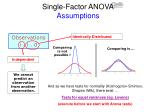Single-Factor ANOVAAssumptions

Observations

i . i . d

Identically Distributed

Comparing is not possible !

Comparing is ...

Independent

We cannot predict an observation from another observation.

And as we have tests for normality (Kolmogorov-Smirnov, Shapiro Wilk), there exist …

Tests for equal variances (eg. Levene)

(execute before we start with Anova tests)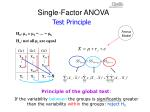Gr1 Gr2 Gr3

X11 X12 X13

...

...

...

Xn3,3

Xn1,1

Xn2,2

X,1

X,2

X,3

X,

X

Single-Factor ANOVA

Test Principle

Anova Model

H0: m1 = m2 = … = mk

Ha: not all µi are equal

Principle of the global test:

If the variability between the groups is significantly greater than the variability within the groups: reject H0Single-Factor ANOVAF-Test
• Null hypothesis: μ1 = μ2 = …μk
• Alternative hypothesis: not all μi are equal
• Test statistic: F = MSG/MSE
• MSG: estimate for the variability among groups (per df)
• MSE: estimate for the variability within groups (per df)
• Decision rule: reject H0 if
• Demonstration:

http://bcs.whfreeman.com/ips5e/Variability between groups

Effect of Indep Var

Mean square =

Sum of Squares / df

MS = SS / df

Fob s= MStreat/MSerror

Reject H0 when Fobs > Fcrit

Variability within groups

Effect of unknown Indep Var or measurement errors.

Reject H0

if p < .05

Residual Variance

Single-Factor ANOVAAnova table

Interpretation of rejection of H0 :

At least one of the group means is different from another group mean.Single-Factor ANOVAExample

So what’s the verdict for the drug effect ?

F value of 3,98 is significant with a p-value of 0,03, which confirms that there is a significant difference in the means.

The F test does not give any specifics about which means are different, only that there is at least one pair of means that is statistically different.

The R-square is the proportion of variation explained by the model.Regression Approach (GLM)Example

From linear regression to the general linear model. Coding scheme for the categorical variable defines the interpretation of the parameter estimates.Regression Approach (GLM)Example - Regressor construction
• Terms are named according to how the regressor variables were constructed.
• Drug[a-placebo] means that the regressor variable is coded as 1 when the level is “a”, - 1 when the level is “placebo”, and 0 otherwise.
• Drug[d-placebo] means that the regressor variable is coded as 1 when the level is “d”, - 1 when the level is “placebo”, and 0 otherwise.
• You can write the notation for Drug[a-placebo] as ([Drug=a]-[Drug=Placebo]), where [Drug=a] is a one-or-zero indicator of whether the drug is “a” or not.
• The regression equation then looks like:

Y = b0 + b1*((Drug=a)-(Drug=placebo)) + b2*(Drug=d)-(Drug=placebo)) + errorRegression Approach (GLM) Example – Parameters and Means
• With this regression equation, the predicted values for the levels “a”, “d” and “placebo” are the means for these groups.
• For the “a” level:

Pred y = 7.9 + -2.6*(1-0) + -1.8*(0-0) = 5.3

For the “d” level:

Pred y = 7.9 + -2.6*(0-0) + -1.8(1-0) = 6.1

For the “placebo” level:

Pred y = 7.9 + -2.6(0-1) + -1.8*(0-1) = 12.3

• The advantage of this coding system is that the regression parameter tells you how different the mean for that group is from the means of the means for each level (the average response across all levels).
• Other coding schemes result in different interpretations of the parameters.Example

CAMELOT Study, JAMA 2004What you will learn

Multifactor Analysis of Variance

ANOVA Basics

Regression versus ANOVA

Model assumptions

Test principle – F-test

GLM approach

Contrasts

Multiple comparisons

Power and Sample size

Diagnostics

Non-parametric alternative

Two-Factor ANOVA

Interaction effect

Analysis of Covariance

Repeated Measures

MANOVASingle-Factor ANOVAContrasts
• Contrasts are often used to analyze (a priori or post-hoc) which group (or factor level) means are different.
• A contrast L is a comparison involving two or more factor level means and is defined as a linear combination of the factor level means µi where the coefficients ci sum to zero.

L = c1µ1+c2µ2+…+ckµk with c1 + c2 + …+ ck = 0

• Examples

L = µ1 - µ2 or L = µ1 - 1/3 µ2 - 1/3 µ3- 1/3 µ4c²j

MSerr ∑

nj

Lobs

Lobs

tobs =

=

SELob

Single-Factor ANOVAContrasts

t-Test for a linear contrast

Hypothesis : H0: L = c1m1+c2m2+…+ckmk = 0 versus H1: L≠0

Estimation of the contrast :

We decide to reject H0 when ׀tobs׀> t N-k, 1-a/2

and accept that L is not equal to zero.Single-Factor ANOVAMultiple comparisons

• In a study we often want to make several comparisons, such as comparing many pairs of means.
• Making multiple comparisons increases the possibility of committing a Type 1 error (declaring something significant that is not in fact significant).
• The more tests you do, the more likely you are to find a significant difference that occurred by chance alone.
• If you are comparing all possible pairs of means in a large ANOVA lay-out, there are many possible tests, and a Type 1 error becomes very likely.Single-Factor ANOVAAdjusting forMultiple comparisons

• There are many methods that modify tests tocontrol for an overall error rate when doing simultaneous comparisons.
• With the method of Bonferroni the overall error rate is divided by the total number of comparisons you want to make. So we test differences between means at a significance level α* = α / c.
• Other multiple comparison methods such as Tukey-Kramer, Sidak or Gabriel are less conservative than Bonferroni. This means that they are more powerful and able to detect smaller differences.Single-Factor ANOVAAdjusting forMultiple comparisons

What can we conclude about the differences between the groups using the comparison circles and the tables on the next slide ?Single-Factor ANOVAAdjusting forMultiple comparisons

Both “a” and “d” appear significantly different than “placebo” with unadjusted tests.

Only Drug “a” is significantly different than “placebo” with the Tukey-Kramer adjusted t-tests

The difference in significance occurs because the quantile that is multiplied with the SE to create a Least Significant Difference has grown from 2.05 to 2.47 between Student’s test and the Tukey-Kramer testSPSS: ANOVA

T test with P-value

no longer 0.05 but

0.05/n of tests performedSingle-Factor ANOVAPower and Sample Size
• Power is the probability of achieving a certain significance when the true means and variances are specified.
• You can use the power concept to help choose a sample size that is likely to give significance for certain effect sizes and variances.
• Power has the following ingredients
• The effect size – that is the seperation of the means
• The standard deviation of the error or the variance
• Alpha, the significance level
• The number of observations, the sample sizeSingle-Factor ANOVAPower and Sample size
• Increase the effect size. Larger differences are easier to detect. For example, when designing an experiment to test a drug, administer as large a difference in doses as possible. Also, use balanced designs.
• Decrease Residual Variance. If you have less noise it is easier to find differences. Sometimes this can be done by blocking or testing within subjects of by selecting a more homogeneous sample.Single-Factor ANOVAPower and Sample size
• Increase the sample size. With larger samples the standard error of the estimate of effect size is smaller. The effect is estimated with more precision. Roughly, the precision increases in proportion to the square root of the sample size.
• Accept less protection. Increase alpha. There is nothing magic about alpha=0.05. A larger alpha lowers the cut-off value. A statistical test with alpha=0.20 declares significant differences more often (and also leads to false conclusions more often).Single-Factor ANOVAPower and Sample size

If you want 90% probability (power) of achieving a significance of 0.01, then the sample size needs to be slightly above 70. For the same power at 0.05 significance, the sample size only needs to be 50ANOVA DiagnosticsResiduals

As in regression, residuals, studentized residuals and studentized deleted residuals are used for diagnosing ANOVA model departures.

Plots of residuals against fitted values, residual dot plots and normal probability plots are helpful in diagnosing following departures from the ANOVA model:

Nonnormality of error terms

Nonconstancy of error variance

Outliers and Influential observations

Nonindependence of error termsANOVA DiagnosticsUnequal Variances

ANOVA assumes the variance is the same for all groups. Various F-based methods test for equality of the variances.

If unequal variances are of concern, you can consider Welch Anova (test in which the observations are weighted by the reciprocals of the esimated variances) or a nonparametric approach or a transformation of the response variable such as the square root or the log.Single-Factor ANOVANonparametric Alternative

Nonparametric procedures do not depend on the distribution of the error term, often the only requirement is that the distribution is continuous.

They are based on the ranks of the data, thus ignoring the spacing information between the data.

Kruskal-Wallis test statistic (h) has an approximate chi-square distribution with k-1 degrees of freedom.

Decision rule: reject H0 ifKruskal-Wallis testExample

What is your conclusion from the Kruskal-Wallis test ? Compare with the Anova results.Analysis of VarianceDemonstration

How to do an analysis of variance with the EXCEL data analysis option ?What you will learn

Multifactor Analysis of Variance

ANOVA Basics

Regression versus ANOVA

Model assumptions

Test principle – F-test

GLM approach

Contrasts

Multiple comparisons

Power and Sample size

Diagnostics

Non-parametric alternative

Two-Factor ANOVA

Interaction effect

Analysis of Covariance

Repeated Measures

MANOVATwo-Factor ANOVAIntroduction
• A method for simultaneously analyzing two factors affecting a response.
• Group effect: treatment group or dose level
• Blocking factor whose variation can be separated from the error variation to give more precise group comparisons: study center, gender, disease severity, diagnostic group, …
• One of the most common ANOVA methods used in clinical trial analysis.
• Similar assumptions as for single-factor anova.
• Non-parametric alternative : Friedman testTwo-Factor ANOVAExample

Do different treatments cause differences in mean response ?

Is there a difference in mean response for males and females ?

Is there an interaction between treatment and gender ?Two-Factor ANOVAInteraction Effect

Two-way Anova allows to evaluate the effect of the individual factors on the response (main effects) and to evaluate interaction effects.

Interaction: treatment affects the response differently depending on the level of the other factor (block).

Source:

Common Statistical Methods for Clinical Research, 1997, Glenn A. WalkerResponse score of subject k in column i androw j

Effect of treatment factor (a levels or i columns )

Interaction effect

Overall Mean

Error or Effect of not measured variables

Effect of blocking factor (b levels or j rows)

Two-Factor ANOVAThe ModelEffect of treatment

Effect of Blocking factor

Error or residual variance

Interaction

Two-Factor ANOVAAnova TableTwo-Factor ANOVAExample (GLM approach)

Questions

How much of the variation of the response is explained by the model ?

What do you conclude from the Lack of Fit test ?

Which of the factors have a significant effect on the response ?

What is the mean response for the males ?

What is the mean response for subjects treated with D ?

What can you do to improve the fit ?Two-Factor ANOVAExample with Interaction

Questions

How much of the variation of the response is explained by the model ?

What can you conclude from the effect test table ?

What is the mean response for males treated with A ?

An interesting phenomenon, which is true only for balanced designs, is that the estimates and SS for the main effects is the same as in the fit without interaction. The F tests are different. Why ? The interaction effect test is identical to the lack-of-fit test in the previous model.Two-Factor ANOVAExample Interaction Plot

The plot visualizes that treatment D has a different effect on mean response of males compared to females.Two-Factor ANOVA

Example with Excel

ANOVA can easily be done with the data analysis module from Excel

ANOVA table from Excel

What can you conclude from this Anova table ?What you will learn

Multifactor Analysis of Variance

ANOVA Basics

Two-Factor ANOVA

Analysis of Covariance

Repeated Measures

MANOVAAnalysis of CovarianceANCOVA
• Method for comparing response means among two or more groups adjusted for a quantitative concomitant variable, or “covariate”, thought to influence the response.
• The response variable is explained by independent quantitative variable(s) and qualitative variable(s).
• Combination of ANOVA and regression.
• Increases the precision of comparison of the group means by decreasing the error variance.
• Widely used in clinical trialsAnalysis of CovarianceThe model
• The covariance model for a single-factor with fixed levels adds another term to the ANOVA model, reflecting the relationship between the response variable and the concomitant variable.
• The concomitant variable is centered around the mean so that the constant µ represents the overall mean in the model.Analysis of CovarianceModel assumptions
• The single factor Ancova model on the previous slide assumes :
• Normality of error terms
• Equality of error variances for different treatments
• Equality of slopes of the different treatment regression lines
• Linearity of regression relation with concomitant variable
• Uncorrelatedness of error termsAnalysis of CovarianceExample

Let’s look again at the response (LBS=bacteria count) of 30 subjects to one of three treatments by adding the continuous effect (LBI=bacteria count at baseline) to the model.Analysis of CovarianceExample

Adding the covariate LBI to the model raises the RSquare form 22.78% to 67.62%.

Lack of fit ? Tests whether anything you have left out of the model is significant.

The parameter estimate for LBI is 0.987, which is not unexpected because the response is the bacteria count, and LBI is the baseline count before treatment. With a coefficient of nearly 1 for LBI, the model is really fitting the difference in bacteria counts.

Drug is no longer significant in this model. How could this be ? The error in the model has been reduced, so it should be easier for differences to be detected.

Or could there be a relationship between LBI and Drug ?Analysis of CovarianceExample

Aha !

The drugs have not been randomly assigned. The toughest cases with the most bacteria tended to be given the “placebo”. The drugs “a” and “d” were given a head start at reducing the bacteria count until LBI was brought into the model.

So it is important to control for all the factors as the significance of one depends on what else is in the model.Analysis of CovariancePrediction Equation

We can calculate the prediction equation from the parameter estimates :

• Predicted LBS =
• 2.695 + 0.987 * LBI + - 1.185 when “a”
• - 1.076 when “d”
• 2.261 when “placebo”Analysis of CovarianceLeverage Plots

Interpretation and conclusions ?Analysis of CovarianceLeast Squares Means

It is not correct to compare raw cell means in the ANCOVA case as raw cell means do not compensate for different covariate values in the model.

Instead we construct predicted values (least squares means, adjusted means), which are the expected value of the observation from some level of the categorical factor when all the other factors (covariates) are set to neutral values.

The role of least-squares means is that they allow comparisons of levels with the control of other factors being held fixed.

We use the prediction equation to calculate these adjusted means.Analysis of CovarianceLeast Squares Means

Least Squares Means for Drug exampleAnalysis of CovarianceInteractions
• When an Ancova model includes a main effect and a covariate regressor, the analysis uses a separate intercept for the covariate regressor for each level of the main effect.
• If the intercepts are different, might not the slopes of the lines also be different? To find out we need a way to capture the interaction of the regression slope with the main effect. This is done by introducing a crossed term, the interaction of Drug and LBI into the model.Analysis of CovarianceInteractions

What is your conclusion ?Analysis of CovarianceInteractions

Illustration of Covariance with Separate Slopes.Example

Powell et al, Circ 2008Example

Powell et al, Circ 2008Example

Powell et al, Circ 2008What you will learn

Multifactor Analysis of Variance

ANOVA Basics

Two-Factor ANOVA

Analysis of Covariance

Repeated Measures

MANOVARepeated-MeasuresBasic concepts
• ‘Repeated-measures’ are measurements taken from the same subject (patient) at repeated time intervals.
• Many clinical studies require:
• multiple visits during the trial
• response measurements made at each visit
• A repeated measures study may involve several treatments or only a single treatment.
• ‘Repeated-measures’ are used to characterize a response profile over time.
• Main research question:
• Is the mean response profile for one treatment group the same as for another treatment group or a placebo group ?
• Comparison of response profiles can be tested with a single F-test.Repeated-MeasuresComparingprofiles

Source:

Common Statistical Methods for Clinical Research, 1997, Glenn A. WalkerRepeated-Measures Designs
• Provide good precision for comparing treatments since between subjects variability is excluded from the residual error.
• Allows to lower the number ofsubjects (patients) needed.
• Disadvantages (if several treatments per subject)
• The order of the treatments might have an effect on the response : order effect
• The preceding treatment(s) might influence the response : carry-over effectRepeated Measures ANOVASingle-Factor Model
• Response may vary
• among treatment groups
• among patients within groups
• among the different measurement times
• Therefore we include in the model
• GROUP (between subject) fixed effect
• SUBJECT (within group) random effect
• TIME (within subject) effect
• GROUP-by-TIME interactionRepeated Measures ANOVAApproaches
• You can analyse repeated measures data with a ‘univariate’ approach using GLM. In addition to normality and variance homogeneity, this approach requires the assumption of ‘compound symmetry’, which means that correlations between each pair of observations are the same. In most repeated measures data, this assumption is not valid.
• A ‘multivariate’ approach can be used to circumvent this problem: repeated measurements become multivariate response vectors (MANOVA).Repeated MeasuresSimpleExample
• Six animals from two species were tracked, and the diameter of the area that each animal wandered was recorded. Each animal was measured four times, once per season.
• Is there a significant difference in mean wandering area between the two species ?Repeated Measures ANOVARandom Effects – Mixed Model

What are your conclusions about the between subjects species effect and the within subjects season effect ?

Prediction FormulaRepeated Measures ANOVACorrelated Measurements – Multivariate Model

Response Profiles

Multi-variate F-tests### Questions?Multi-factor ANOVATake-home messages
• Use ANOVA to compare group means; to analyze the effect of one or more qualitative variables on a continuous response variable.
• Use ANCOVA to analyze concomitantly the effect of a quantitative independent variable (covariate).
• Significances of differences in means are tested with the F-statistic, comparing between-group variation with within-group variation.
• Always use graphics to look at the data and to investigate themodel assumptions.
• Carefully analyze the interaction effects.
• Analyse repeated measures by comparing profile plots using the GLM or the multivariate MANOVA approach.### And now a brief break…For further slides on these topics please feel free to visit the metcardio.org website:http://www.metcardio.org/slides.html